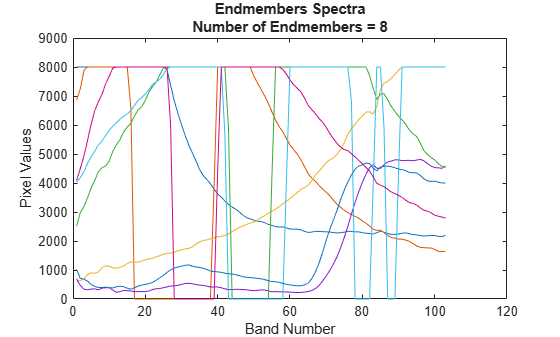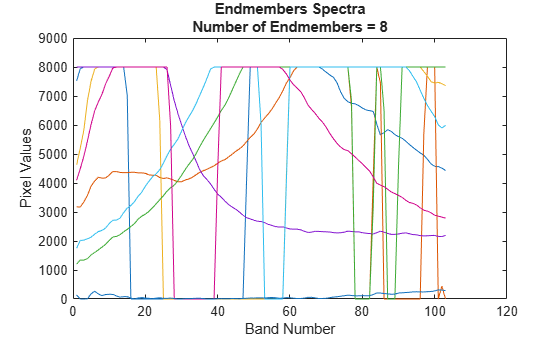nfindr

Extract endmember signatures using N-FINDR

Description

example

endmembers = nfindr(inputData,numEndmembers) extracts endmember signatures from hyperspectral data cube by using the N-finder (N-FINDR) algorithm. numEndmembers is the number of endmember signatures to be extracted using N-FINDR algorithm. For more information about the N-FINDR method, see Algorithms.

example

endmembers = nfindr(inputData,numEndmembers,Name,Value) specifies options using one or more name-value pair arguments in addition to the input arguments in the previous syntax. Use this syntax to set the options for number of iterations and dimensionality reduction.

Note

This function requires the Image Processing Toolbox™ Hyperspectral Imaging Library. You can install the Image Processing Toolbox Hyperspectral Imaging Library from Add-On Explorer. For more information about installing add-ons, see Get and Manage Add-Ons.

Examples

collapse all

Read a hyperspectral data into the workspace.

hcube = hypercube('paviaU.hdr');

Find the number of spectrally distinct endmembers present in the hyperspectral data cube by using countEndmembersHFC function.

numEndmembers = countEndmembersHFC(hcube,'PFA',10^-7);

Compute the endmembers using the N-FINDR method. By default, the nfindr function uses maximum noise fraction (MNF) transform for preprocessing. The default value for number of iterations is 3 times the number of estimated endmembers.

endmembers = nfindr(hcube.DataCube,numEndmembers);

Plot the endmembers of the hyperspectral data.

figure
plot(endmembers)
xlabel('Band Number')
ylabel('Pixel Values')
ylim([0 9000])
title({'Endmembers Spectra',['Number of Endmembers = ' num2str(numEndmembers)]});Read a hyperspectral data into the workspace.

hcube = hypercube('paviaU.hdr');

Find the number of spectrally distinct endmembers present in the hyperspectral data cube by using countEndmembersHFC function.

numEndmembers = countEndmembersHFC(hcube,'PFA',10^-7);

Compute the endmembers using the N-FINDR method. Specify the value for number of iterations as 1000. Select principal component analysis (PCA) as the dimensionality reduction method for preprocessing.

endmembers = nfindr(hcube.DataCube,numEndmembers,'NumIterations',1000,'ReductionMethod','PCA');

Plot the endmembers of the hyperspectral data.

figure
plot(endmembers)
xlabel('Band Number')
ylabel('Pixel Values')
ylim([0 9000])
title({'Endmembers Spectra',['Number of Endmembers = ' num2str(numEndmembers)]});Input Arguments

collapse all

Input hyperspectral data, specified as an 3-D numeric array or a hypercube object. If the input is a hypercube object, then the function reads the hyperspectral data from its DataCube property.

The hyperspectral data is an numeric array of size M-by-N-by-C. M and N are the number of rows and columns in the hyperspectral data respectively. C is the number of spectral bands in the hyperspectral data.

Data Types: single | double | int8 | int16 | int32 | int64 | uint8 | uint16 | uint32 | uint64

Number of endmembers to extract, specified as a positive scalar integer. The value must be in the range [1 C]. C is the number of spectral bands in the input hyperspectral data.

Data Types: single | double | int8 | int16 | int32 | int64 | uint8 | uint16 | uint32 | uint64

Name-Value Arguments

Specify optional comma-separated pairs of Name,Value arguments. Name is the argument name and Value is the corresponding value. Name must appear inside quotes. You can specify several name and value pair arguments in any order as Name1,Value1,...,NameN,ValueN.

Example: nfindr(cube,7,'NumIterations',100,'Method','None')

Number of iterations, specified as a positive scalar integer. The default value is 3P. P is the number of endmember signatures to be extracted. The computation time of the algorithm increases with the increase in the number of iterations.

Data Types: single | double | int8 | int16 | int32 | int64 | uint8 | uint16 | uint32 | uint64

Dimensionality reduction method, specified as one of these values:

• 'MNF' — To perform dimensionality reduction by using the maximum noise fraction (MNF) method. This is the default.

• 'PCA' — To perform dimensionality reduction by using the principal component analysis (PCA) method.

If you specify this argument, the function first reduces the spectral dimension of the input data by using the specified method. Then, it computes the endmember signatures from the reduced data.

Data Types: char | string

Output Arguments

collapse all

Endmember signatures, returned as a matrix of size C-by-P and datatype same as the datatype of the input hyperspectral data.

Data Types: single | double | int8 | int16 | int32 | int64 | uint8 | uint16 | uint32 | uint64

Algorithms

N-FINDR is an iterative approach for finding the endmembers of a hyperspectral data. The method assumes that the volume of a simplex formed by the endmembers (purest pixels) is larger than any other volume defined by any other combination of pixels . The steps involved are as follows:

1. Compute principal component bands and reduce the spectral dimensionality of the input data by using MNF or PCA. The number of principal component bands to be extracted is set equal to the number of endmembers to be extracted. The endmembers are extracted from the principal component bands.

2. Randomly select n number of pixel spectra from the reduced data as initial set of endmembers.

3. For iteration 1, denote the initial set of endmembers as $\text{\hspace{0.17em}}\left\{{\text{e}}_{1}^{\left(1\right)},\text{\hspace{0.17em}}\text{\hspace{0.17em}}{\text{e}}_{2}^{\left(1\right)},\text{\hspace{0.17em}}\text{\hspace{0.17em}}\cdots \text{\hspace{0.17em}},\text{\hspace{0.17em}}\text{\hspace{0.17em}}{\text{e}}_{p}^{\left(1\right)}\right\}$.

Consider the endmembers as vertices of a simplex and compute the volume by using

$\text{\hspace{0.17em}}V\left({\text{E}}^{\left(1\right)}\right)\text{\hspace{0.17em}}=\text{\hspace{0.17em}}|\mathrm{det}\left({\text{E}}^{\left(1\right)}\right)|$

where ${\text{E}}^{\left(1\right)}\text{\hspace{0.17em}}=\text{\hspace{0.17em}}\text{\hspace{0.17em}}\left[\begin{array}{l}1\text{\hspace{0.17em}}\text{\hspace{0.17em}}\text{\hspace{0.17em}}\text{\hspace{0.17em}}\text{\hspace{0.17em}}\text{\hspace{0.17em}}\text{\hspace{0.17em}}\text{\hspace{0.17em}}\text{\hspace{0.17em}}\text{\hspace{0.17em}}\text{\hspace{0.17em}}\text{\hspace{0.17em}}1\text{\hspace{0.17em}}\text{\hspace{0.17em}}\text{\hspace{0.17em}}\text{\hspace{0.17em}}\text{\hspace{0.17em}}\text{\hspace{0.17em}}\text{\hspace{0.17em}}\text{\hspace{0.17em}}\cdots \text{\hspace{0.17em}}\text{\hspace{0.17em}}\text{\hspace{0.17em}}\text{\hspace{0.17em}}\text{\hspace{0.17em}}\text{\hspace{0.17em}}\text{\hspace{0.17em}}1\\ {\text{e}}_{1}^{\left(1\right)}\text{\hspace{0.17em}}\text{\hspace{0.17em}}\text{\hspace{0.17em}}\text{\hspace{0.17em}}{\text{e}}_{2}^{\left(1\right)}\text{\hspace{0.17em}}\text{\hspace{0.17em}}\text{\hspace{0.17em}}\text{\hspace{0.17em}}\cdots \text{\hspace{0.17em}}\text{\hspace{0.17em}}\text{\hspace{0.17em}}\text{\hspace{0.17em}}{\text{e}}_{p}^{\left(1\right)}\end{array}\right]\text{\hspace{0.17em}}\text{\hspace{0.17em}}\text{\hspace{0.17em}}$.

4. For iteration 2, Select a new pixel spectra r, such that $\text{\hspace{0.17em}}\text{r}\text{\hspace{0.17em}}\notin \left\{{\text{e}}_{1}^{\left(1\right)},\text{\hspace{0.17em}}\text{\hspace{0.17em}}{\text{e}}_{2}^{\left(1\right)},\text{\hspace{0.17em}}\text{\hspace{0.17em}}\cdots \text{\hspace{0.17em}},\text{\hspace{0.17em}}\text{\hspace{0.17em}}{\text{e}}_{p}^{\left(1\right)}\right\}$.

5. Replace each endmember in the set with r and compute the volume of the simplex V(E(2)).

6. Replace the ith endmember in the set with r, if the computed volume V(E(2)) is greater than V(E(1)). This results in an updated set of endmembers. For example, if i = 2, the new set of endmembers derived at the end of the second iteration is $\text{\hspace{0.17em}}\left\{{\text{e}}_{1}^{\left(2\right)},\text{\hspace{0.17em}}\text{\hspace{0.17em}}{\text{e}}_{2}^{\left(2\right)}=\text{\hspace{0.17em}}\text{\hspace{0.17em}}\text{r},\text{\hspace{0.17em}}\text{\hspace{0.17em}}\cdots \text{\hspace{0.17em}},\text{\hspace{0.17em}}\text{\hspace{0.17em}}{\text{e}}_{p}^{\left(2\right)}\right\}$.

7. For each iteration, select a new pixel spectra r and repeat steps 5 and 6. Each iteration results in an update set of endmembers. The iteration ends when the total number of iterations reaches the specified value NumIterations.

 Winter, Michael E. “N-FINDR: An Algorithm for Fast Autonomous Spectral End-Member Determination in Hyperspectral Data.” Proc. SPIE Imaging Spectrometry V 3753, (October 1999): 266–75. https://doi.org/10.1117/12.366289.